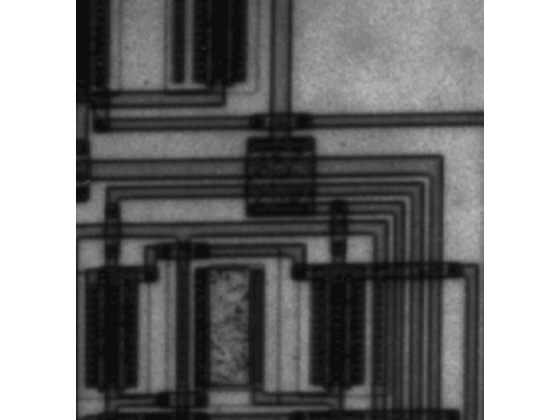# houghpeaks

Identify peaks in Hough transform

## Syntax

peaks = houghpeaks(H,numpeaks)
peaks = houghpeaks(H,numpeaks,Name,Value)

## Description

example

peaks = houghpeaks(H,numpeaks) locates peaks in the Hough transform matrix, H, generated by the hough function. numpeaks specifies the maximum number of peaks to identify. The function returns peaks a matrix that holds the row and column coordinates of the peaks.
peaks = houghpeaks(H,numpeaks,Name,Value) controls aspects of the operation using name-value pair arguments.

## Examples

collapse all

Read image into workspace.

I = imread('circuit.tif');

Create binary image.

BW = edge(imrotate(I,50,'crop'),'canny');

Create Hough transform of image.

[H,T,R] = hough(BW);

Find peaks in the Hough transform of the image and plot them.

P = houghpeaks(H,2); imshow(H,[],'XData',T,'YData',R,'InitialMagnification','fit'); xlabel('\theta'), ylabel('\rho'); axis on, axis normal, hold on; plot(T(P(:,2)),R(P(:,1)),'s','color','white');## Input Arguments

collapse all

Hough transform matrix, specified as a numeric array. The rows and columns correspond to rho and theta values. Use the hough function to create a Hough transform matrix.

Data Types: double

Maximum number of peaks to identify, specified as a positive integer.

Data Types: double

### Name-Value Arguments

Specify optional pairs of arguments as Name1=Value1,...,NameN=ValueN, where Name is the argument name and Value is the corresponding value. Name-value arguments must appear after other arguments, but the order of the pairs does not matter.

Before R2021a, use commas to separate each name and value, and enclose Name in quotes.

Example: P = houghpeaks(H,2,'Threshold',15);

Minimum value to be considered a peak, specified as a nonnegative number.

Data Types: double

Size of the suppression neighborhood, specified as a 2-element vector of positive odd integers. The suppression neighborhood is the neighborhood around each peak that is set to zero after the peak is identified. The default value of NHoodSize is the smallest odd values greater than or equal to size(H)/50. The dimensions of NHoodSize must be smaller than the size of the Hough transform matrix, H.

Data Types: double

Hough transform theta values, specified as a numeric vector returned by the hough function. Each element of the vector specifies the theta value for the corresponding column of the output matrix H. houghpeaks uses the theta values specified for peak suppression. Use the hough function to create a Hough transform matrix.

Note

If you specify the 'Theta' parameter as input to the hough function, you must specify the theta parameter with the houghpeaks function. Use the theta output value from the hough function as the theta input value for houghpeaks. Otherwise, peak suppression can result in unexpected results.

Data Types: double

## Output Arguments

collapse all

Row and column coordinates of found peaks, returned as a Q-by-2 matrix. The value Q can range from 0 to numpeaks.

## Version History

Introduced before R2006a